/

### Blending Independent Components and Principal Components Analysis 2.7 Complexity pursuit

Next page

2.7          Complexity pursuit

Most signals measured within a physical system can be expected to be a mixture of statistically independent source signals. The most parsimonious explanation for the complexity of an observed signal is thus that it consists of a mixture of simpler signals, each from a different source. Underpinning this is the assumption that a mixture of independent source signals is typically more complex than the simplest (i.e. least complex) of its constituent source signal. This complexity conjecture underpins the idea of complexity pursuit.

One simple measure of complexity is predictability. If each value of a signal is relatively easy to predict from previous signal values then we might characterise the signal as having low complexity. Conversely, if successive values of a signal are independent of each other then prediction is in principle impossible and we might characterise such a signal as having a high complexity.

Stone (2004), when discussing this technique, focuses on minimising Kolmogorov complexity and defines a measure,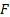, of temporal predictability for a given set of signal mixtures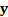and weights,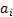, applied to these signal mixtures as follows: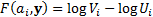Here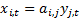,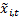is a suitable exponentially weighted moving average of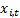, i.e.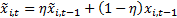for some suitable (perhaps predefined) value of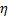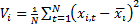corresponds to the overall variance of the given linear combination and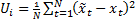corresponds to the extent to which it is well predicted by its previous values (assuming here a first order autoregressive dependency).

Complexity pursuit has certain advantages and disadvantages over ICA and projection pursuit. Unlike ICA it does not appear explicitly to include an a priori model for the signal pdfs, but seems only to depend on the complexity of the signal. It ought therefore to be able to extract signals with different pdf types. It also does not ignore signal structure, e.g. its temporal nature if it is a time series. Conversely, ‘complexity’ is a less obviously well-defined concept than independence or non-normality. For example, the prescription introduced by Stone (2004) and described above seems to be very heavily dependent on ‘lack of complexity’ being validly equated with signals exhibiting strong one-period autodependency.